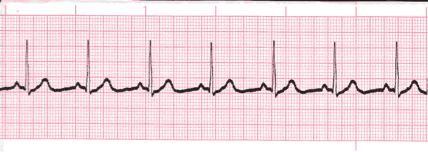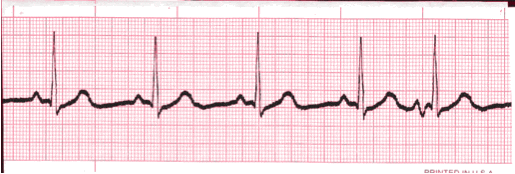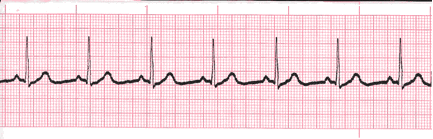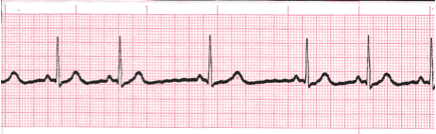# Step 2 - Heart Rate Regular - Cardiac Rhythms.

## Regular Rhythms

• If the rhythm varies by less than two small boxes, then the rhythm is considered regular.
• The heart rate determination technique used will be the 1500 technique.
• Starting at the beginning of the tracing through the end, measure from one R wave to the next R wave (ventricular assessment), then P wave to P wave (atrial assessment), then count the number of small boxes between each and divide that number into 1500.
• This technique will give you the most accurate heart rate when analyzing regular heart rhythms. You may include ½ of a small box i.e. 37.5/1500 = 40 bpm (don’t forget to round up or down if a portion of a beat is included in the answer).## Irregular Rhythms

• If the rhythm varies by two small boxes or more, the rhythm is considered “irregular”.
• The heart rate determination technique used for irregular rhythms will be the “six-second technique”.
• Simply count the number of complete cardiac complexes in six seconds and multiply by ten.## Practice 1

Analyze this tracing. What is the heart rate?Analyze this tracing. What is the heart rate?

Reveal Answer Regular Rhythm – 1500 technique – 22 small boxes divided into 1500 = 68.18. Rate: 68 bpm

## Practice 2Analyze this tracing. What is the heart rate?

Reveal Answer Irregular Rhythm – Six-second technique – 5 complete complexes. Rate: 50 bpm.

## Authors and Reviewers

Authored by Thomas O'Brien
Medically reviewed by Dr. Jonathan Keroes, MD, Cardiology
Last Update: 11/8/2020

An error has occurred. This application may no longer respond until reloaded. Reload 🗙